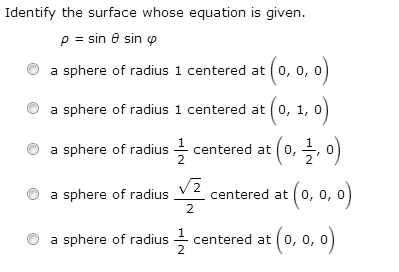# [SOLVED] Identify the surface whose equation is given. (Spherical Coordinate Calculus)?

edited

Identify the surface whose equation is given. (Spherical Coordinate Calculus)?

Identify the surface when given:

ρ = sin θ sin φ

I'm having trouble figuring this out. The only thing that looks like it might be close to something is the fact that y = ρ sinθ sin φ, but even then I am not sure how to identify the surface that way.Thanks for the help, guys!

selected by

## This is the Best Answer for Identify the surface whose equation is given. (Spherical Coordinate Calculus)?

Multiply both sides by ρ:

==> ρ^2 = ρ sin θ sin φ.

Now, we can directly convert to rectangular coordinates:

x^2 + y^2 + z^2 = y, which is a sphere.

To get this into standard form, complete the square:

x^2 + (y^2 - y) + z^2 = 0

==> x^2 + (y^2 - y + 1/4) + z^2 = 1/4

==> x^2 + (y - 1/2)^2 + z^2 = (1/2)^2, which has center (0, 1/2, 0) and radius 1/2.

I hope this helps!

Lorem ipsum dolor sit amet, consectetur adipiscing elit, sed do eiusmod tempor incididunt ut labore et dolore magna aliqua. Ut enim ad minim veniam, quis nostrud exercitation ullamco laboris nisi ut aliquip ex ea commodo consequat. Duis aute irure dolor in reprehenderit in voluptate velit esse cillum dolore eu fugiat.